# DRAW ME LIKE ONE OF YOUR FRENCH GIRLS: Neural artistic style-transfer

Date:
Category:
Machine Learning

One of the coolest applications of image processing with deep convolutional neural nets is the transfer of artistic style. By this, I mean suppose you had a nice Instagram selfie, and you wanted Van Gogh to paint you a painting of your selfie, and show it to all your friends. Unfortunately Van Gogh is dead, but his style lives on in his paintings. His brush textures, color palette, curve style and characteristic shapes all constitute an intuitive sense of style that humans would recognize.

Neural style-transfer synthesizes a third image from two sources: a content image and a style image. The content image provides the base content (such as your selfie) and the style image provides the style reference (Van Gogh or Pollock). Style features such as colors, textures, edges and shapes are extracted and combined with the content image in a natural way, e.g. eyes and lips are shaded consistently with the hues and textures found in the style image and original content image shapes are preserved. These style features are encoded by cross-correlation of neural net outputs in Gram matrices, and to synthesize a style transfer means generating an output image that minimizes the distance between each element of the Gram matrices of the content image and the style image. More on this later.

App companies like SnapChat and Prisma are ahead on the style-transfer game to provide advanced effects filters to photos. Extensions of the the style-transfer concept are far reaching and extend to for example reinterpreting Chopin’s Prelude in E-Minor in the style of Bossa Nova (here’s a non-ML attempt). Improved algorithms for style-transfer have been developed, but let’s go back to the original pioneering work of Gatys et. al and see how this style-extraction and synthesis was first accomplished with an intuitive and minimal math explanation.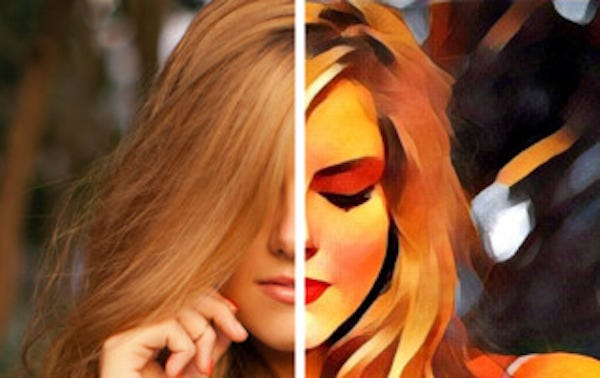A demo of Prisma’s style-transfer filter

The basis of Gatys et. al’s original neural style-transfer is the convolutional neural net or convnet in ML parlance. The famous VGG-19 convnet was used by Gatys et. al in their pioneering work. If you are not familiar with convnets, here’s a good primer on the subject. The main takeaway about convnets is that they learn progressively more abstract image features along each block. A block is a collection of layers and each layer has a feature map. A feature map is the output of a convolutional layer after it applies the convolve and activation transforms. The layers in successive blocks progress from learning colors to edges to shapes. VGG-19 is a fairly simple yet powerful convnet used for image classification.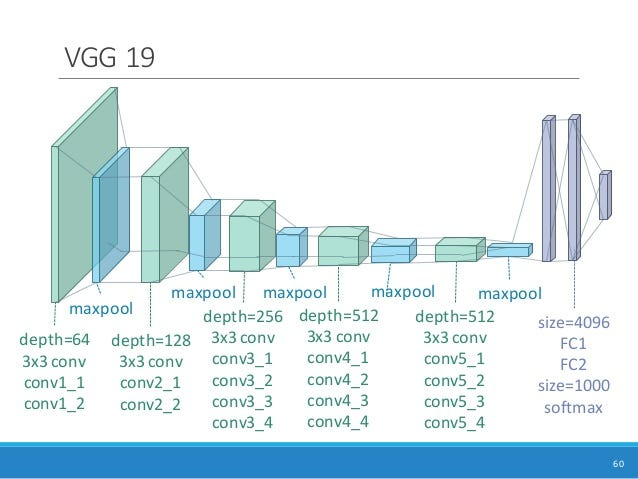Architecture of the VGG-19 Network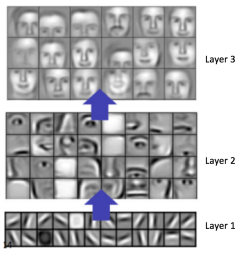Illustration of progressively higher-level features learned by a convnet

The VGG-19 net has a total of 5 blocks, 5 maxpooling layers and 3 fully-connected layers. The interesting part is we do not actually train the neural net. We use a pre-trained VGG-19 model (such as keras.applications.vgg19) and feed the content image, style image and initially random synthesized image to the net. Then we tap off the feature maps in each block and completely ignore the fully connected layers. Let’s call the synthesized image or the result of the style-transfer x, the content image and the style image s. All of the input images are tensors of shape (width, height, color depth). The synthesized image is an that minimizes the objective function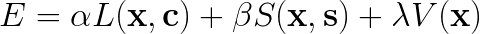In practice the above objective is optimized using a quasi-Newton method. Note that the style-transfer algorithms learns an optimal x* that minimizes E, the parameters αβ, and λ are not updated (they are manually tuned) and the VGG-19 weights are not updated either. α and β are the mixture parameters, i.e. α determines how much the original content image should be preserved and β determines how strongly the style elements should be mixed into xλ is a regularization constant to adjust the effect of V(x), which is typically the total variation function. The total variation regularization promotes smoothness in the output image especially along edges of shapes.

The L(xc) function is the content loss. The content loss function is the euclidean distance between VGG-19 feature maps when is fed to the net, and then when is fed. Feature maps can be thought of as 2D matrices or collapsed to 1D vectors and then the content loss is simply the euclidean distance between two feature vectors. This content loss term in the objective function is a strong incentive to maintain the abstract image features of the original image in the output image x. In the style-transfer algorithm at the end of the article, the feature map used was from the conv5_2 layer which basically is the output layer for and contains high level features such as complete color, texture, edge and shape information.

Next, The S(xs) function is called the style loss is analogous to content loss but instead of calculating a direct euclidean distance between feature maps, it calculates the euclidean distance between feature map Gram matrices for each VGG-19 block — there are five Gram matrices in the example code at the end. A Gram (or Grammian) matrix in this context is defined as the following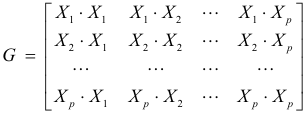Where each X is a different 1D feature map vector corresponding to different layers from the same block. The Gram matrix contains the unshifted cross-correlation (the dot product between two vectors is equivalent to unshifted cross-correlation) between each feature map vector (i.e. an entire feature map such as layer conv3_1 with layer conv3_2) in a block. If you recall, the feature map contains a high level presentation of features such as colors, texture, edges and shapes. A cross-correlation of feature maps on an image with respect to itself gives a measure of which features in an image occur together, and these correlated features constitute the style of an image intuitively. In the style-transfer algorithm the Gram matrix for layers chosen by the designer (usually a few from each block) is computed for inputs and s and then the euclidean distance between each element in the Gram matrices and the sGram matrices are computed. There is an additional weighing parameter (tuned by the designer) that weighs the contribution from each Gram matrix distance calculation. Note the Gram matrix cross-correlation is calculated between feature maps for one image x or against itself, not between x and s. In essence, the style of each image in and s is seen by the net when we feed them forward. We then extract these styles and synthesize them by minimizing the style loss or the dissimilarity in styles between and sA recent article by Li et al. gives a theoretical basis for S(xs) that minimizes the distance between probability distributions of the style features.

Okay, so that was a bit of math, neural nets and some signal processing. Hopefully, you can see that the style transfer concept is not so bad. The overall procedure for neural style-transfer is as follows:

1. Initialize randomly, preferably with the same image size as c.
2. Compute the losses L(xc), S(xs) and V(x)
3. Optimize w.r.t. the losses in step 2 using an algorithm such as L-BFGS(note L-BFGS returns an iterative estimate of the optimal x*)
4. Using the returned by step 3, repeat steps 2–4 until thoroughly satisfied

The following python script is a slightly modified version of the one found in the Keras examples repo. You can experiment with tuning the content_weight, style_weight and tv_weight parameters to get different blends of your style and content images (extreme style weights give more shape distortions). As well, using different feature_layers can lead to some interesting results.

``````
'''Neural style transfer with Keras.
Run the script with:
```
python neural_style_transfer.py path_to_your_base_image.jpg path_to_your_reference.jpg prefix_for_results
```
e.g.:
```
python neural_style_transfer.py img/tuebingen.jpg img/starry_night.jpg results/my_result
```
Optional parameters:
```
--iter, To specify the number of iterations the style transfer takes place (Default is 10)
--content_weight, The weight given to the content loss (Default is 0.025)
--style_weight, The weight given to the style loss (Default is 1.0)
--tv_weight, The weight given to the total variation loss (Default is 1.0)
```
It is preferable to run this script on GPU, for speed.
# Details
Style transfer consists in generating an image
with the same "content" as a base image, but with the
"style" of a different picture (typically artistic).
This is achieved through the optimization of a loss function
that has 3 components: "style loss", "content loss",
and "total variation loss":
- The total variation loss imposes local spatial continuity between
the pixels of the combination image, giving it visual coherence.
- The style loss is where the deep learning keeps in --that one is defined
using a deep convolutional neural network. Precisely, it consists in a sum of
L2 distances between the Gram matrices of the representations of
the base image and the style reference image, extracted from
different layers of a convnet (trained on ImageNet). The general idea
is to capture color/texture information at different spatial
scales (fairly large scales --defined by the depth of the layer considered).
- The content loss is a L2 distance between the features of the base
image (extracted from a deep layer) and the features of the combination image,
keeping the generated image close enough to the original one.
# References
- [A Neural Algorithm of Artistic Style](http://arxiv.org/abs/1508.06576)
'''

from __future__ import print_function
from scipy.misc import imsave
import numpy as np
from scipy.optimize import fmin_l_bfgs_b
import time
import argparse

from keras.applications import vgg19
from keras import backend as K

parser = argparse.ArgumentParser(description='Neural style transfer with Keras.')
help='Path to the image to transform.')
help='Path to the style reference image.')
help='Prefix for the saved results.')
help='Number of iterations to run.')
help='Content weight.')
help='Style weight.')
help='Total Variation weight.')

args = parser.parse_args()
base_image_path = args.base_image_path
style_reference_image_path = args.style_reference_image_path
result_prefix = args.result_prefix
iterations = args.iter

# these are the weights of the different loss components
total_variation_weight = args.tv_weight
style_weight = args.style_weight
content_weight = args.content_weight

# dimensions of the generated picture.
img_nrows = height
img_ncols = width

# util function to open, resize and format pictures into appropriate tensors

def preprocess_image(image_path):
img = img_to_array(img)
img = np.expand_dims(img, axis=0)
img = vgg19.preprocess_input(img)
return img

# util function to convert a tensor into a valid image

def deprocess_image(x):
if K.image_data_format() == 'channels_first':
x = x.reshape((3, img_nrows, img_ncols))
x = x.transpose((1, 2, 0))
else:
x = x.reshape((img_nrows, img_ncols, 3))
# Remove zero-center by mean pixel
x[:, :, 0] += 103.939
x[:, :, 1] += 116.779
x[:, :, 2] += 123.68
# 'BGR'->'RGB'
x = x[:, :, ::-1]
x = np.clip(x, 0, 255).astype('uint8')
return x

# get tensor representations of our images
base_image = K.variable(preprocess_image(base_image_path))
style_reference_image = K.variable(preprocess_image(style_reference_image_path))

# this will contain our generated image
if K.image_data_format() == 'channels_first':
combination_image = K.placeholder((1, 3, img_nrows, img_ncols))
else:
combination_image = K.placeholder((1, img_nrows, img_ncols, 3))

# combine the 3 images into a single Keras tensor
input_tensor = K.concatenate([base_image,
style_reference_image,
combination_image], axis=0)

# build the VGG16 network with our 3 images as input
# the model will be loaded with pre-trained ImageNet weights
model = vgg19.VGG19(input_tensor=input_tensor,
weights='imagenet', include_top=False)

# get the symbolic outputs of each "key" layer (we gave them unique names).
outputs_dict = dict([(layer.name, layer.output) for layer in model.layers])

# compute the neural style loss
# first we need to define 4 util functions

# the gram matrix of an image tensor (feature-wise outer product)

def gram_matrix(x):
assert K.ndim(x) == 3
if K.image_data_format() == 'channels_first':
features = K.batch_flatten(x)
else:
features = K.batch_flatten(K.permute_dimensions(x, (2, 0, 1)))
gram = K.dot(features, K.transpose(features))
return gram

# the "style loss" is designed to maintain
# the style of the reference image in the generated image.
# It is based on the gram matrices (which capture style) of
# feature maps from the style reference image
# and from the generated image

def style_loss(style, combination):
assert K.ndim(style) == 3
assert K.ndim(combination) == 3
S = gram_matrix(style)
C = gram_matrix(combination)
channels = 3
size = img_nrows * img_ncols
return K.sum(K.square(S - C)) / (4. * (channels ** 2) * (size ** 2))

# an auxiliary loss function
# designed to maintain the "content" of the
# base image in the generated image

def content_loss(base, combination):
return K.sum(K.square(combination - base))

# the 3rd loss function, total variation loss,
# designed to keep the generated image locally coherent

def total_variation_loss(x):
assert K.ndim(x) == 4
if K.image_data_format() == 'channels_first':
a = K.square(x[:, :, :img_nrows - 1, :img_ncols - 1] - x[:, :, 1:, :img_ncols - 1])
b = K.square(x[:, :, :img_nrows - 1, :img_ncols - 1] - x[:, :, :img_nrows - 1, 1:])
else:
a = K.square(x[:, :img_nrows - 1, :img_ncols - 1, :] - x[:, 1:, :img_ncols - 1, :])
b = K.square(x[:, :img_nrows - 1, :img_ncols - 1, :] - x[:, :img_nrows - 1, 1:, :])
return K.sum(K.pow(a + b, 1.25))

# combine these loss functions into a single scalar
loss = K.variable(0.)
layer_features = outputs_dict['block5_conv2']
base_image_features = layer_features[0, :, :, :]
combination_features = layer_features[2, :, :, :]
loss += content_weight * content_loss(base_image_features,
combination_features)

feature_layers = ['block1_conv1', 'block2_conv1',
'block3_conv1', 'block4_conv1',
'block5_conv1']
for layer_name in feature_layers:
layer_features = outputs_dict[layer_name]
style_reference_features = layer_features[1, :, :, :]
combination_features = layer_features[2, :, :, :]
sl = style_loss(style_reference_features, combination_features)
loss += (style_weight / len(feature_layers)) * sl
loss += total_variation_weight * total_variation_loss(combination_image)

# get the gradients of the generated image wrt the loss

outputs = [loss]
else:

f_outputs = K.function([combination_image], outputs)

if K.image_data_format() == 'channels_first':
x = x.reshape((1, 3, img_nrows, img_ncols))
else:
x = x.reshape((1, img_nrows, img_ncols, 3))
outs = f_outputs([x])
loss_value = outs
if len(outs[1:]) == 1:
else:

# this Evaluator class makes it possible
# to compute loss and gradients in one pass
# while retrieving them via two separate functions,
# "loss" and "grads". This is done because scipy.optimize
# requires separate functions for loss and gradients,
# but computing them separately would be inefficient.

class Evaluator(object):

def __init__(self):
self.loss_value = None

def loss(self, x):
assert self.loss_value is None
self.loss_value = loss_value
return self.loss_value

assert self.loss_value is not None
self.loss_value = None

evaluator = Evaluator()

# run scipy-based optimization (L-BFGS) over the pixels of the generated image
# so as to minimize the neural style loss
x = preprocess_image(base_image_path)

for i in range(iterations):
print('Start of iteration', i)
start_time = time.time()
x, min_val, info = fmin_l_bfgs_b(evaluator.loss, x.flatten(),
print('Current loss value:', min_val)
# save current generated image
img = deprocess_image(x.copy())
fname = result_prefix + '_at_iteration_%d.png' % i
imsave(fname, img)
end_time = time.time()
print('Image saved as', fname)
print('Iteration %d completed in %ds' % (i, end_time - start_time))
```
```

here you have it, a working example of the neural style-transfer code and an intuitive explanation of how this style transfer works. Since Gatys et al.numerous improved algorithms have been developed some of them way faster than this one, but in essence the same concepts of styles in neural net feature maps and optimizing over some sort of similarity measure between features is retained. As always, I hope you have fun making your own style transfers, and let me know if you have any questions or issues with the code.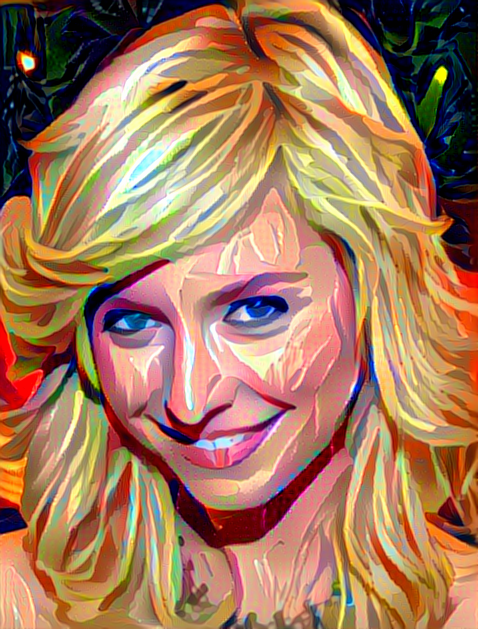“So, can you make me one of those cool painting style photos too?”